### Home > INT3 > Chapter 10 > Lesson 10.1.3 > Problem10-45

10-45.
1. Lorna is looking at the series 5 + 3 + 10 + 8 + 15 + … + 400 + 398. Homework Help ✎

2. “The series definitely isn’t arithmetic, but it does have a pattern,” Lorna tells her team, “I wonder if we can still calculate the sum of the series.”

1. What patterns do you see in Lorna’s series? Describe them as completely as you can.

2. Do you agree with Lorna’s statement that this series is not arithmetic? Justify your conclusion. Do you think it is still possible to calculate a sum for the series?

3. “I think I see it!” Lorna exclaims. “I think we can separate the terms into the two different arithmetic series shown below, kind of like unzipping a zipper.” Her work is shown below.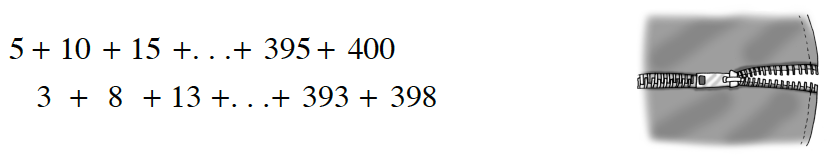How can you use Lorna’s two series to compute the sum of the original series? State the sum and describe your process.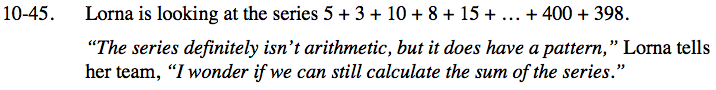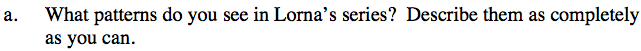Look at the first four terms. There are repetitive decreases of 2 and increases of 7.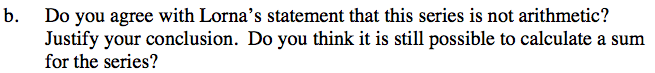Can you write an equation for the terms of the sequence in the form t(n) = an + b?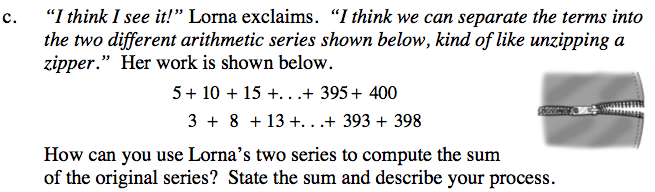Find the sum of these two new series independently, and add the two sums together to find the original sum.

32, 240